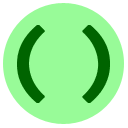# 第1部分：避免进行重复计算

`1, 1, 2`

`1, 1, 2, 3, 5, 8, 13, ...`• def Fibonacci(n):
• return Fibonacci(n-1) + Fibonacci(n-2)
• if (n==1 or n==2):
• return 1
• else:

## 答案• sequence = [0, 1, 1] # Fibonacci(0) is 0, Fibonacci(1) and Fibonacci(2) are 1
• return sequence[n]
• sequence.append(sequence[i-1] + sequence[i-2])
• for i in range(3, n+1):
• def Fibonacci(n):

# 第2部分：避免计算不重要的事情，一次也不要

Python中如何测试一个数字是否是质数？我们之前讲过如何测试整除性：

`N % D == 0  # will be True if D is a divisor of N, False otherwise`

## 主意和参数

`A*B == N`

`N = A*B > sqrt(N)*sqrt(N) > N`

## 最后的练习题这是CS Circles网站最后的练习题。恭喜完成所有课程的同学！查看resources page，里面有些建议告诉你接下来可以学什么。希望你可以从中找到乐趣，编写出好程序！祝你好运！